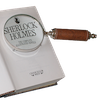#### You may also like### Number Detective

Follow the clues to find the mystery number.### Six Is the Sum

What do the digits in the number fifteen add up to? How many other numbers have digits with the same total but no zeros?### ABC

In the multiplication calculation, some of the digits have been replaced by letters and others by asterisks. Can you reconstruct the original multiplication?

# Calculator Bingo

##### Age 7 to 11Challenge Level

This is a two player game. You will each need a calculator and you will need one 0-9 dice.

The object of the game is to be the first to get exactly to zero.

How to play:

1. Enter a 7-digit number into your calculator. You may use the same digit more than once.
2. Throw the dice. If this digit appears in the number each player has on their calculator, they can change that digit to zero by subtracting the appropriate number using the calculator keys.
3. If you subtract the correct amount, this digit will automatically change to zero. If you are incorrect, you will be allowed another chance.
4. For every throw of the dice, you can only remove one digit from your calculator. So if you have, for example, four 3's you must wait for 3 to come up four times.
5. Throw the dice again. Each player can again change that digit to zero if it appears in their number.
6. The first to reach exactly zero is the winner.